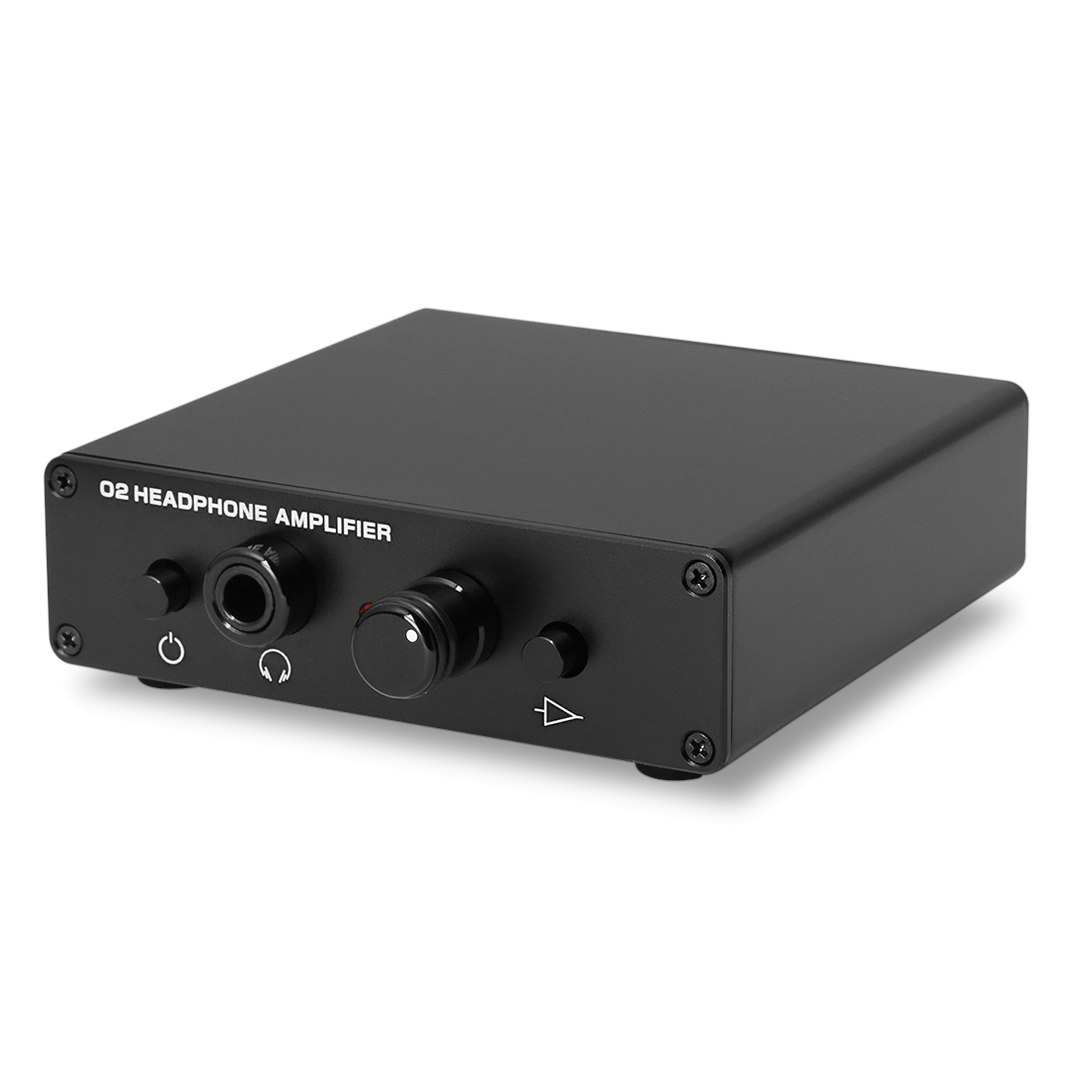Showing 1 of 1900 conversations about:Massdrop Objective 2 Headphone Amp: Desktop Edition
Please explain about gain. Standard Gain (2.5x Low, 6.5x High) - is this means that it basically has 3 gain options: 1) without any gain (is it 1.0x?) 2) 2.5x Low 3) 6.5x High
And what about Medium Gain (1.0x Low, 3.3x High) - is this means that only 2 modes available: 1) without any gain (is it 1.0x?) 2) 1.0x Low 3) 3.3x High So are (1) and (2) the same? (both are: 1.0x)
Aug 12, 2017
There is not a "no gain" option. Gain is always in use, so both choices only have two gain options each. If you are going to be using this with a dedicated DAC, go with the medium option. If you are planning to use it with a phone or DAP I would suggest the standard option.
Aug 12, 2017
1X gain is 'unity gain.' The level coming out is the same as the level coming in. 1V of input signal = 1V of output signal.
Since we inconveniently measure output in terms of 'watts', it's hard to figure out what's going on from the specs alone.
"0 (zero) gain" would mean no sound at all from the output. I would not pay money for that. ;)
On my O2, the gain switch is 2-position. I have the standard 2.5X Low and 6.5X High gain settings, using Fostex T50RP headphones (65 ohms). I would only choose the 1.0X/3.3X gain option if I was using very sensitive, low impedance headphones or earbuds with 32 ohm or lower impedance.
Aug 12, 2017
Thanks for the explanations. So, you advise to go with Standard model (2.5X Low and 6.5X High)?
Aug 12, 2017
"0 gain" is a little unclear. It could mean 0x gain, which would be no sound, but is more likely to mean 0dB gain, which is the same as 1.0x gain.
Which gain option to choose has just as much (if not more) to do with your source voltage as it does with your headphone impedance. If your only sources are going to be 2v mains powered DACs or components, then choose medium gain. If you want to use portable sources with lower voltage output, then standard gain might be beneficial.
Aug 12, 2017
I am totally confused. Just read what Nwavguy wrote about gain: <<< Gain is usually expressed as a factor (ratio), such as 4X, or in decibels (dB) such as 12 dB. In this case, 4X is the same as 12 dB and simply means with the volume control set to maximum, the output voltage of the amplifier will be four times higher than the input voltage. If you put 1 volt in you will get 4 volts out (assuming you don't exceed the maximum output capability) >>> So what it means in the case of Moderate Gain model at Low Gain mode, which is 1x? So if volume control is not set to maximum at Low Gain mode it should reduce input voltage - this looks really stupid.
Aug 14, 2017
Yes you've got it right :) At 1.0x gain the output voltage will be lower than the input voltage when the volume control is at less than maximum. Think about it, when the volume control is at minimum you would expect the output voltage to be zero. The gain just determines what the voltage will be at maximum volume, and the volume control varies from zero up to maximum output voltage (which will be input voltage x gain factor).
Aug 14, 2017
Thanks for your explanations. Now I got to the main summary. I just realized, please correct if I am wrong. For example, HD650 (HD6XX) ideally need enough gain to get up to at least 2 volts from whatever your source can manage (typically at least 4X or about 12 dB). So 4X can be receives as a sum of Source input + Amp output. This means if Standard Gain O2 Amp can give at Low Gain mode max 2.5x, then Source should provide the rest, which is 1.5x (2.5x+1.5x=4x). If the Source in not powerful enough then I have to switch the Amp into High Gain mode, which can supply upto 6.0x, so this 6.0x will cover itself all requirements for HD650 (HD6XX). In case of Moderate Gain Model: at Low Gain mode it can provide only 1.0x maximum, which means the Source should give the rest 3.0x And at at High Gain mode Amp can add 3.5x max , so the Source should provide at least 0.5x.
Is this correct?
Aug 14, 2017
You seem to be getting confused about the gain factor. When gain is expressed as 1x or 2.5x or 4x, it's simply a multiplier, indicating how many times the input voltage will be increased. So if your input voltage is 2v and your gain factor is 2.5x, then your max output voltage will be 2v x 2.5 = 5v.
Gain can also be expressed in dB. A gain factor of 4x is approximately the equivalent of +12 dB.
Typically the lowest input voltage you're likely to come across will be around 0.5v (some portable devices) so in order to get up to the 2v max output recommended for the HD650 you'd need at least 4x gain.
But mains powered sources (DACs and typical HiFi components) will commonly output at around 2v, so you might not need any gain at all (1x or 0dB gain) and you certainly don't want to be using 6.5x gain!
Aug 14, 2017